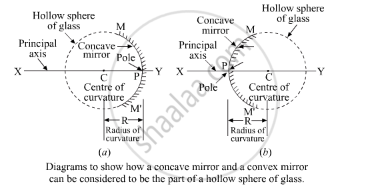Share

# Define (A) Centre of Curvature (B) Radius of Curvature (C) Pole (D) Principal Axis, and (E) Aperture, of a Spherical Mirror with the Help of a Labelled Diagram - CBSE Class 10 - Science

ConceptReflection of Light Spherical Mirrors

#### Question

Define (a) centre of curvature (b) radius of curvature (c) pole (d) principal axis, and (e) aperture, of a spherical mirror with the help of a labelled diagram

#### Solution

(a) Centre of curvature (C) – It is the centre of the sphere from which the mirror is formed.
(b) Radius of curvature (R) – It is the radius of the sphere from which the mirror is formed.
R = PC

(c) Pole (P) – Pole is the centre of the curved mirror surface, MM’.
(d) Principal axis – It is the line joining P and C.
(e) Aperture – It is the effective length of the mirror, i.e. MM’.Is there an error in this question or solution?

#### Video TutorialsVIEW ALL 

Solution Define (A) Centre of Curvature (B) Radius of Curvature (C) Pole (D) Principal Axis, and (E) Aperture, of a Spherical Mirror with the Help of a Labelled Diagram Concept: Reflection of Light - Spherical Mirrors.
S